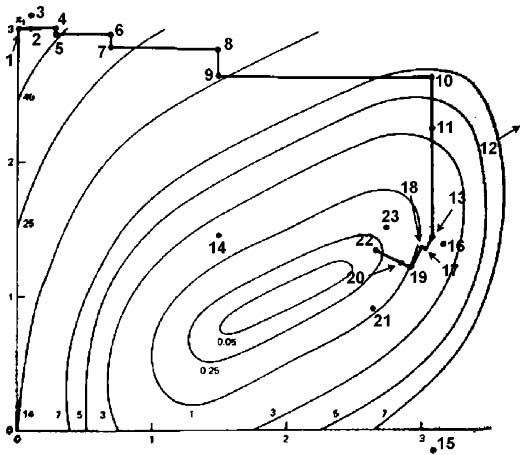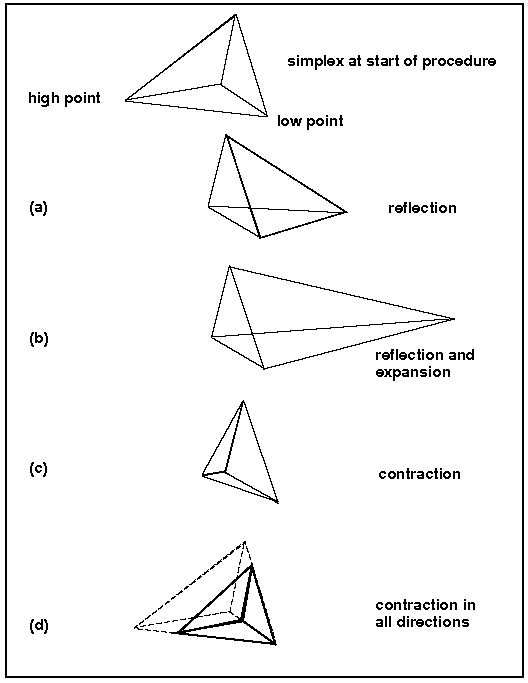#### Rosenbrock algorithm

The Rosenbrock method is a 0th order search algorithm and it does not require gradient of the target function. Only simple evaluations of the objective function are used. Yet, this algorithm approximates a gradient search thus combining advantages of 0th order and 1st order strategies.In the first iteration, it is a simple 0th order search in the directions of the base vectors of an n-dimensional coordinate system (in the figure above n=2). In the case of a success, which is an attempt yielding a new minimum value of the target function, the step width is increased, while in the case of a failure it is decreased and the opposite direction will be tried (see points 1 to 16 in the figure above). Once a success has been found and exploited in each base direction, the coordinate system is rotated in order to make the first base vector point into the direction of the gradient (the points 13,16,&17 are defining the new base in the figure above). Now all step widths are initialized and the process is repeated using the rotated coordinate system (points 16 to 23). The creation of a new rotated coordinate system is usually done using a Gram-Shmidt orthogonalization procedure. In extreme cases, this algorithm becomes numerically instable. This instabillity can lead to a premature ending of the optimization algorithm. The Gram-Shmidt orthogonalization procedure can also become very time consuming when the dimension n of the search space increases (the complexity of the procedure is n^3). The new orthogonalization procedure of J.R.Palmer solves these issues.